# 42. Trapping Rain Water

Reference: LeetCode
Difficulty: Hard

## Problem

Given n non-negative integers representing an elevation map where the width of each bar is 1, compute how much water it is able to trap after raining.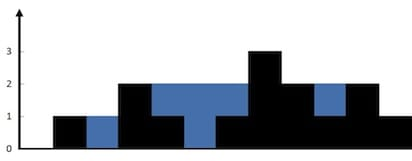Example:

## Analysis

Wrong Idea: One pointer to detect increase or decrease. My mind was influenced by the solution to the longest mountain in an array.

A tricky test case: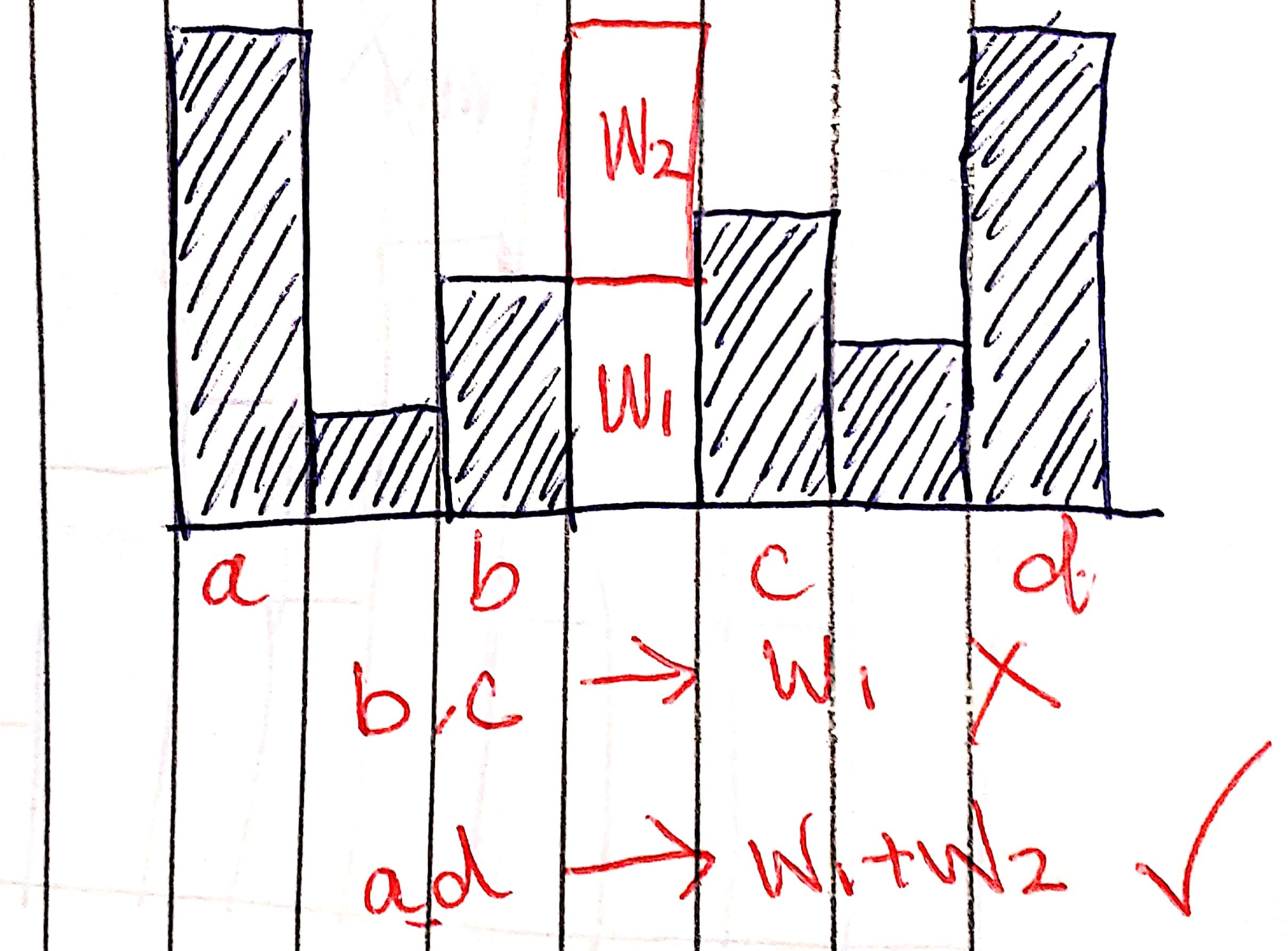The correct answer is w1 + w2. To compute the amount, we can’t just go by the bars b and c; instead, we must consider a and d with a broader perspective.

Come up with the brute-force first!

### Brute-Force

For each position i in height[0...n-1], calculate how much water it contains. The amount of water can be computed by min(leftMax, rightMax) - height[i]. where leftMax is the highest left bar in height[0...i-1] and rightMax is the highest right bar in height[i+1...n-1]. What does it mean? No Picture You Say J8~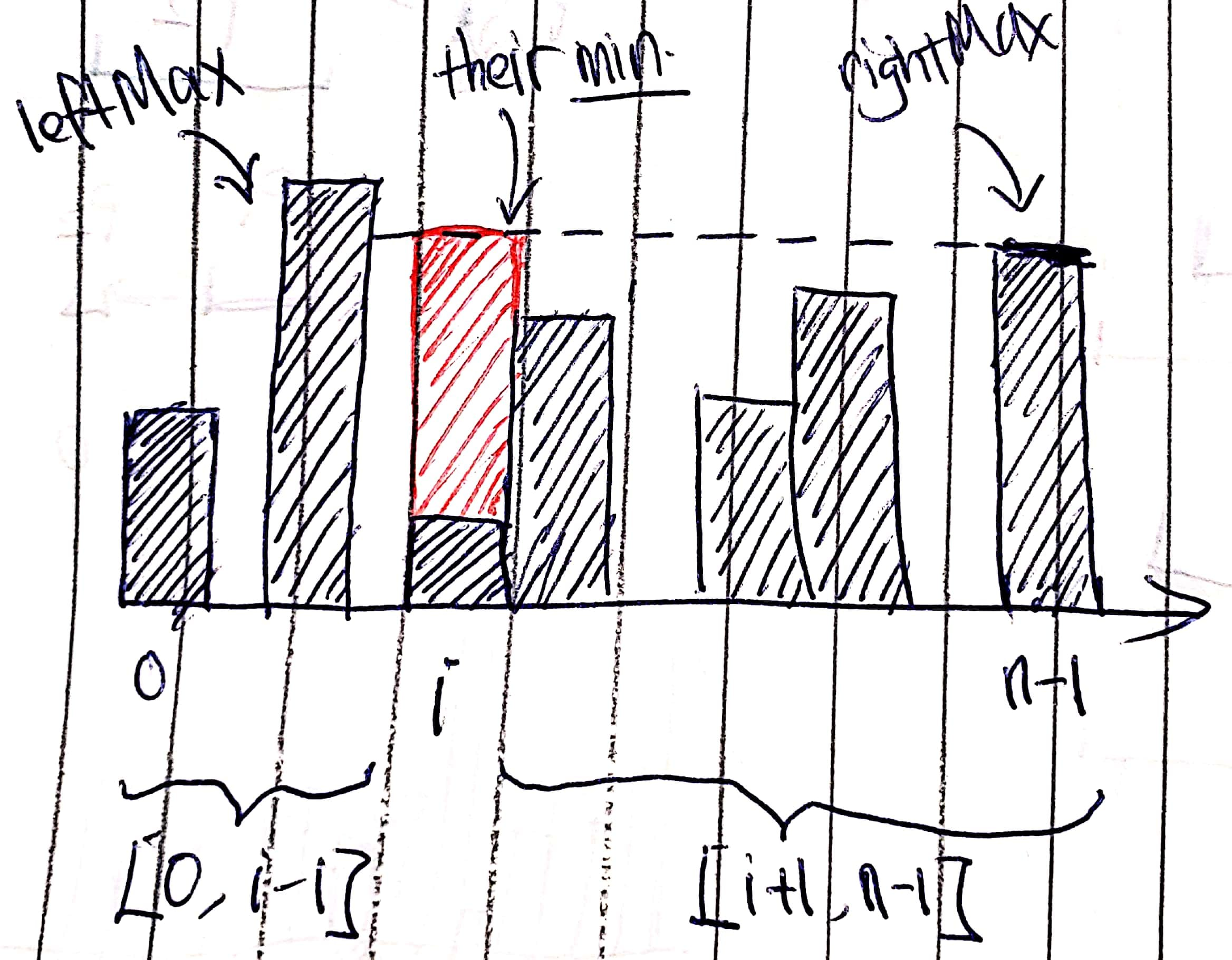Note:

• When i equals 0 and n - 1, the amount must be 0 since min(leftMax, rightMax) is 0.
• Notice that if the amount of water is negative, we just skip it. It happens when height[i] is greater than min(leftMax, rightMax), which means no water is stored on it.

Time: $O(N^2)$ since computing leftMax and rightMax in each round takes $O(N)$.
Space: $O(1)$

### DP (pre-compute)

Based on the brute-force approach, we can pre-compute all leftMax and rightMax in advance, which reduces time complexity to $O(N)$.

We denote leftMax[i] as the highest bar in height[0...i] and rightMax[i] as the highest bar in height[i...n-1].

Note: Before pre-computation, leftMax and rightMax[n - 1] are each initialized by height and height[n - 1]. By doing this, the code in the loop is cleaner.

Time: $O(N)$
Space: $O(N)$

### Divide and Conquer

We can also solve this problem by divide-and-conquer approach.

Initially, leftMax is height and rightMax is height[n - 1]. We recursively compute the amount of trapping water in the problem subTrap[1, n - 2] with leftMax and rightMax.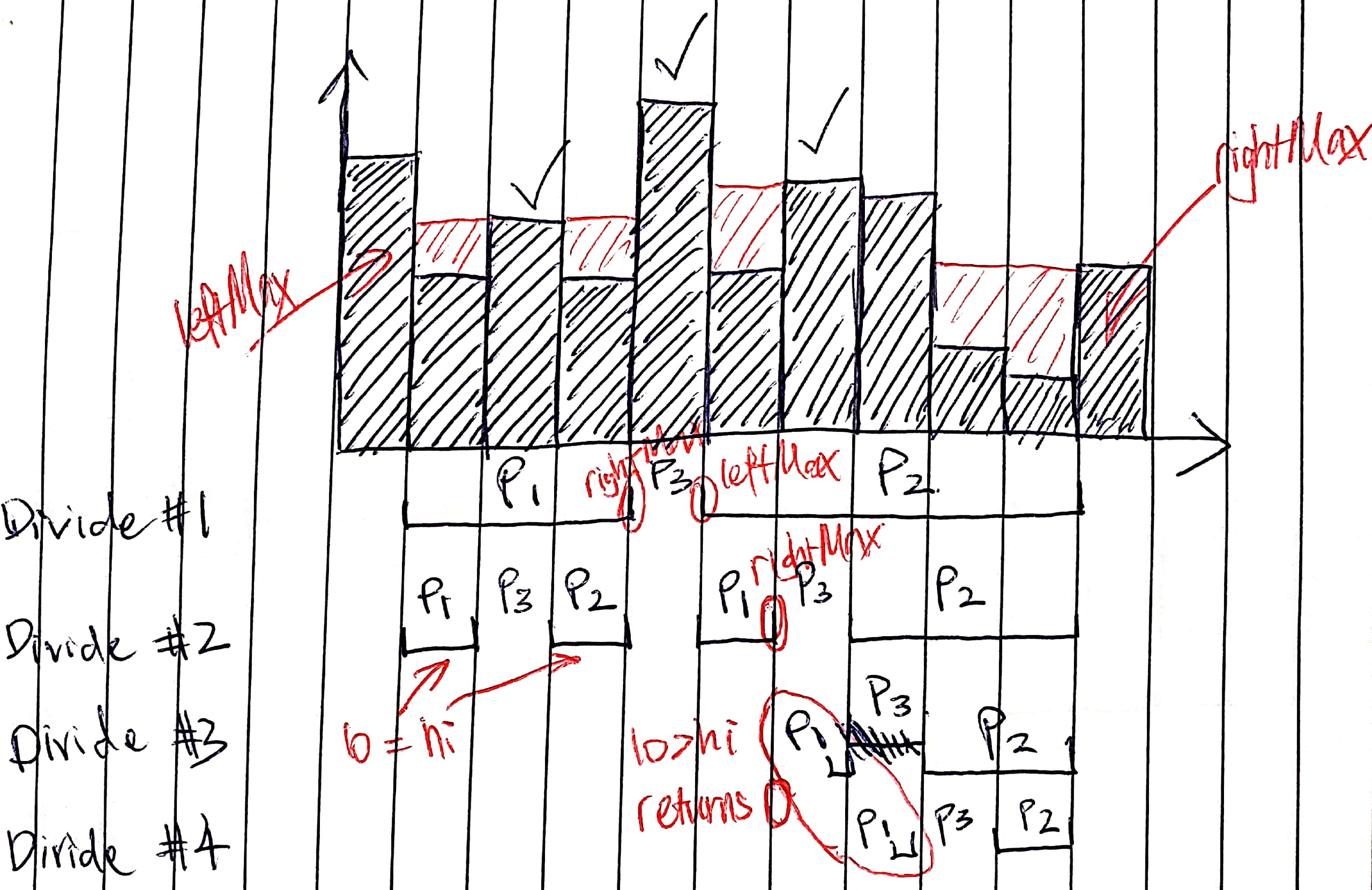Divide: We use findMax to find the highest bar i in height[lo, hi], then divide into three subproblems p1, p2, and p3.
Conquer: We compute p1 and p2 recursively. p3 can be computed by height[i] - min(leftMax, rightMax) (remember to skip negative results).
Combine: Returns p1 + p2 + p3.

In each step, we update rightMax for the left subproblem p1 and update leftMax for the right subproblem p2 if they are greater than height[findMax[i]].

Base Case: When there is one position (lo == hi), just solve it as we did for p3. If there is no place (lo > hi), returns 0.

Time:

• Best case: $O(N\log{N})$ where $T(N) = 2T(N/2) + O(N)$
• Worst case: $O(N^2)$ where $T(N) = T(N-1) + O(N)$

Space:

• Best case: $O(\log{N})$
• Worst case: $O(N)$

It depends on how the problem is divided into two subproblems by the highest bar.

Note: Time complexity can be reduced to $O(N)$ if the divide step findMax() takes $O(1)$ (pre-compute), no matter how the problem is divided.

### Two Pointers (no extra space, clever)

Note: We have leftMax and rightMax that record the largest heights lo and hi have seen so far.

As in DP approach, instead of computing each leftMax and rightMax separately, we can actually consider one bar at each time as our min(leftMax, rightMax) since we only care about the bar with a smaller height.

We denote two pointers lo and hi starting from two ends of the array. In the loop, we update leftMax and rightMax first.

If the current leftMax is less than rightMax, we can correctly compute the water at lo, but not the water at hi.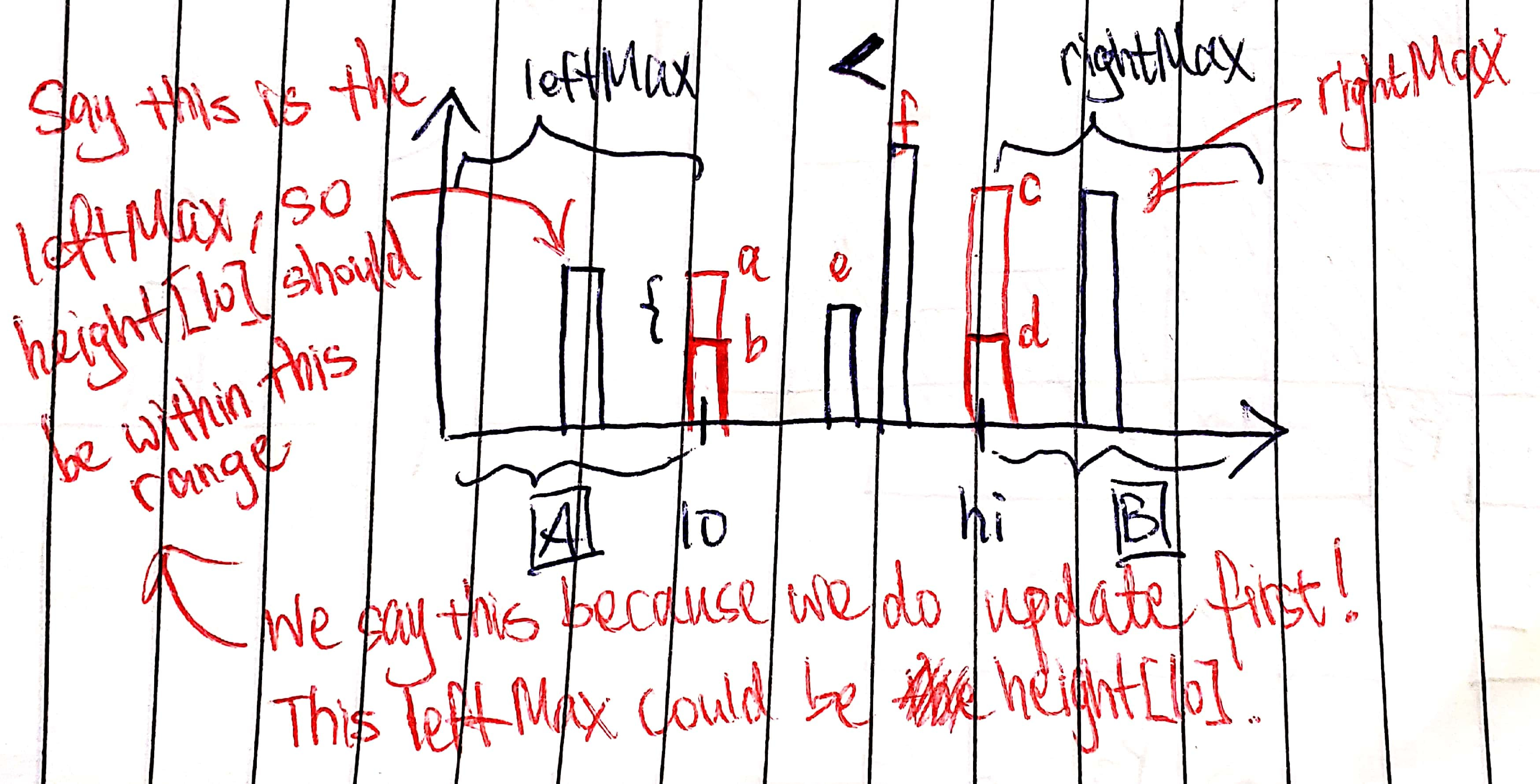Let’s examine the case leftMax < rightMax. We assume a leftMax in height[0, lo]. Since we do update first, height[lo] should be less than or equal to leftMax (it means that leftMax could have been just updated by height[lo]).

Since leftMax < rightMax, the amount of water at lo can be determined at this time, no matter what the heights between [lo + 1, hi - 1] are. For example, if there is an e higher than b but lower than leftMax, it still works because there is rightMax on the right that can eliminate the effect of e. In terms of f, it is more obvious that it is right. (it is hard to explain well T_T)

However, it does not always hold true if we compute the amount of water at hi in this case (leftMax < rightMax). Consider d that is less than rightMax:

• If we use leftMax to compute the amount (leftMax - d), there could be an f that gives a larger result (min(f, rightMax) - d).

• If we use rightMax to compute the amount (rightMax - d), there could be an e that gives a smaller result (min(e, rightMax) - d if e > leftMax or min(leftMax, rightMax) - d if e < leftMax).

Here is the code:

Time: $O(N)$
Space: $O(1)$

### Two Pointers (my first attempt)

Here is the solution I first came up with… It just uses two pointers to keep track of two bars and compute the water by levels constrained by the minimum height of two bars. (a bit similar to Skyline problem…)

Left/right pointers are both aiming for higher bars when they move on to the center. It is not intuitive because each time we should not add computed amount of water (minus wateredHt in the code).

How could I write such a solution instead of brute-force at first?See a better approach below.

Time: $O(N^2)$
Space: $O(1)$

CommentJunhao Wang
a software engineering cat